Applies to TestComplete 14.20, last modified on September 11, 2019Keyword test operations can be set up in two ways:

• Using dialogs that provides visual access to the operation parameters.

• Using in-place editors of the Keyword Test editor.

An operation can also support both approaches let the users use any one they prefer.

This topic explains how you can implement in-place editing support for custom keyword test operations created with script extensions. Before you proceed, we recommend that you read the Customizing KeywordTest Editor Contents topic to learn about specifying the operation data to be displayed in the Keyword Test editor. To learn how to display edit forms for the operation, see Creating the Operation Setup Routine.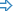Making a Keyword Test editor’s Column EditableImplementing In-Place Editor FunctionalityValidating User Input

Making a Keyword Test editor’s Column Editable

By default, the information on custom operations displayed in the Keyword Test editor is read-only. To make data in a particular column editable, you need to extend column definitions in the description.xml file and writing additional script code. The entire procedure consists of the following steps:

1. In the description.xml file, locate or add the Column element corresponding to the desired column.

2. Set the Editable attribute of that Column element to True.

3. Specify the column’s in-place editor type in the EditorType attribute. The following editor types are available:

Editor Type Description Example
Text A text box. This is the default editor type. It is typically used in the Description column.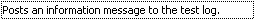Combo A combo box. This editor type is typically used in the Operation column.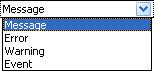Button A text box with a number of embedded buttons. Some operations use this editor type in the Item column.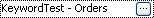Parameters A text box that displays the list of operation parameters. The parameters are listed in the same order as they are defined in the script extension’s description.xml file. The text box contains an ellipsis button, a click on which invokes the Operation Parameters dialog where the user can modify the parameter values. This editor type is typically used in the Value column.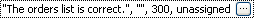If you omit the Editable attribute, the column will use the Text editor.

4. In the script file that contains the operation’s implementation, create routines that will handle events of the in-place editor. You can find detailed information about writing the event handlers code in the subsequent sections of this topic.

5. Attach the event handlers to the editor by defining the event handlers in the description.xml file. This is done by adding the child Event elements to the Column element.

Implementing In-Place Editor Functionality

Implementing Basic Functionality

In-place editors used in the Keyword Test editor raise various events. They occur when the editors are activated, when they request the data to display, when their value is changed, when the editing is finished, and so on. By handling these events, you control the editor state and behavior.

All types of in-place editors, except for the Parameters editor (see below), have a number common events. They are:

• StartEditing - Occurs when the user activates the in-place editor.

• GetEditValue - Occurs when the editor requests the value to display.

• SetEditValue - Occurs when the editor reports its new value.

• HasChanges - Occurs when the editor checks if the value in the in-place editor has been modified.

• ApplyChanges - Occurs when the user finishes the editing and accepts the changes made.

To implement basic functionality of an in-place editor, you need to handle its StartEditing, GetEditValue, SetEditValue and ApplyChanges events. The common implementation of these events is shown in the example below. The example assumes that the in-place editor is used to modify the operation’s MyField field:

JScript

var EditValue;

function MyOperation_StartEditing(Data)
{
EditValue = Data.MyField;
}

function MyOperation_GetEditValue(Data)
{
return EditValue;
}

function MyOperation_SetEditValue(Data, Value)
{
EditValue = Value;
}

function MyOperation_ApplyChanges(Data)
{
Data.MyField = EditValue;
}

VBScript

Dim EditValue

Sub MyOperation_StartEditing(Data)
EditValue = Data.MyField
End Sub

Function MyOperation_GetEditValue(Data)
MyOperation_GetEditValue = EditValue
End Function

Sub MyOperation_SetEditValue(Data, Value)
EditValue = Value
End Sub

Sub MyOperation_ApplyChanges(Data)
Data.MyField = EditValue
End Sub

In this example, the value in the in-place editor is stored in a global script variable - EditValue. The event handlers manipulate this variable to provide feedback between the operation and the in-place editor:

• The StartEditing event handler initializes the EditValue variable with the value being edited (in this example - the operation’s field).

• The GetEditValue event handler reports the current value of EditValue to the in-place editor whenever it requests the value to display.

• The SetEditValue event handler receives the new value for EditValue from the in-place editor.

• Finally, the ApplyChanges event handler copies the value of the EditValue variable to the operation’s field once the user closes the in-place editor accepting the changes.

Implementing Text Editor

A custom keyword test operation uses the Text editor in a particular column of the Keyword Test editor if the EditorType attribute of the corresponding Column element is set to Text. The text editor raises only basic events listed in the previous section. For an example of how a Text editor can be implemented, see the code in the previous section as well.

Implementing a Combo Editor

Combo editors drop down a list of items from which a user can choose. To use this editor in a particular column of the Keyword Test editor, set the EditorType attribute of the corresponding Column element to Combo (see the example below).

Combo editors have events common to all in-place editors (see Implementing Basic Functionality), plus a number of specific events:

• GetCount - Obtains the number of items in the combo box. Default is 0.

• GetItem - Obtains a combo box item by index. Default is an empty string.

• AllowInputText - Checks whether the users are allowed to manually enter values in the combo box. Default is False.

So, to implement a Combo editor support in a custom keyword test operation, you need to handle common events as well as the mentioned specific events. Let’s see how you can do this.

Suppose, you are creating an operation that would close or terminate a process and you want to let the users select the desired action -- Close or Terminate -- from the combo box in the Operation column. Below is the code that manages the Combo in-place editor. In this example, the user’s choice is saved to the operation’s internal Action field.

description.xml

...
<KDTOperation Name="My Operation">
<Data>
<Field Name="Action" />
</Data>
<Columns>
<Column Name="Item" Value="My Operation" />
<Column Name="Operation" GetValue="MyOperation_GetOperation" Editable="True" EditorType="Combo">
<Event Name="GetCount" Routine="MyOperation_Operation_GetCount" />
<Event Name="ApplyChanges" Routine="MyOperation_Operation_GetItem" />
<Event Name="StartEditing" Routine="MyOperation_Operation_StartEditing" />
<Event Name="GetEditValue" Routine="MyOperation_GetEditValue" />
<Event Name="SetEditValue" Routine="MyOperation_SetEditValue" />
<Event Name="ApplyChanges" Routine="MyOperation_Operation_ApplyChanges" />
</Column>
</Columns>
</KDTOperation>
...

JScript

var Actions = ["Close", "Terminate"];
var EditValue;

// Returns the value to display in the Operation column
function MyOperation_GetOperation(Data)
{
return Data.Action;
}

// Returns the number of items in the combo box in the Operations column
function MyOperation_Operation_GetCount(Data, Index)
{
return Actions.length;
}

// Returns a combo box item by index
function MyOperation_Operation_GetItem(Data, Index)
{
return Actions[Index];
}

// Basic event handlers

function MyOperation_Operation_StartEditing(Data)
{
EditValue = Data.Action;
}

function MyOperation_GetEditValue(Data)
{
return EditValue;
}

function MyOperation_SetEditValue(Data, Value)
{
EditValue = Value;
}

function MyOperation_Operation_ApplyChanges(Data)
{
Data.Action = EditValue;
}

VBScript

Dim Actions, EditValue
Actions = Array ("Close", "Terminate")

' Returns the value to display in the Operation column
Function MyOperation_GetOperation(Data)
MyOperation_GetOperation = Data.Action
End Function

' Returns the number of items in the combo box in the Operations column
Function MyOperation_Operation_GetCount(Data, Index)
MyOperation_GetCount = UBound(Actions) + 1
End Function

' Returns a combo box item by index
Function MyOperation_Operation_GetItem(Data, Index)
MyOperation_GetItem = Actions(Index)
End Function

' Basic event handlers

Sub MyOperation_Operation_StartEditing(Data)
EditValue = Data.Action
End Sub

Function MyOperation_GetEditValue(Data)
MyOperation_GetEditValue = EditValue
End Function

Sub MyOperation_SetEditValue(Data, Value)
EditValue = Value
End Sub

Sub MyOperation_Operation_ApplyChanges(Data)
Data.Action = EditValue
End Sub

Implementing a Button Editor

Button editors are text boxes with a number of embedded buttons that perform various helper actions. To use this editor in a particular column of the Keyword Test editor, set the EditorType attribute of the corresponding Column element to Button (see the example below).

Button editors raise common events (see Implementing Basic Functionality) as well as a number of specific events:

• GetButtonCount - Obtains the number of buttons embedded into the editor. Default is 0.

• GetButtonImage - Obtains the image for the specified button. By default, buttons display an ellipsis.

• OnButtonClick - Occurs when a button is clicked.

So, to implement the Button editor support in a custom keyword test operation, you need to handle both common events and the mentioned specific events. The example below demonstrates how this can be done.

The sample operation from this example uses the Button in-place editor in the Value column, which displays the value of the operation’s Value field. The in-place editor has one button that one button that, when clicked, resets the editing value to the default one.

description.xml

...
<KDTOperation Name="My Operation">
<Data>
<Field Name="Value" />
</Data>
<Events>
<Event Name="OnCreate" Routine="MyOperation_OnCreate" />
</Events>
<Columns>
<Column Name="Item" Value="My Operation" />
<Column Name="Value" GetValue="MyOperation_GetValue" Editable="True" EditorType="Button">
<Event Name="GetButtonCount" Routine="MyOperation_Value_GetButtonCount"/>
<Event Name="GetButtonImage" Routine="MyOperation_Value_GetButtonImage"/>
<Event Name="OnButtonClick" Routine="MyOperation_Value_OnButtonClick"/>
<Event Name="StartEditing" Routine="MyOperation_Value_StartEditing"/>
<Event Name="GetEditValue" Routine="MyOperation_GetEditValue"/>
<Event Name="SetEditValue" Routine="MyOperation_SetEditValue"/>
<Event Name="HasChanges" Routine="MyOperation_Value_HasChanges"/>
<Event Name="ApplyChanges" Routine="MyOperation_Value_ApplyChanges"/>
</Column>
</Columns>
</KDTOperation>
...

JScript

// The default value of the Value field
var DEFAULT_VALUE = "test";

// Initializes the values of operation's fields
function MyOperation_OnCreate(Data)
{
Data.Value = DEFAULT_VALUE;
}

// Returns the text to be displayed in the Keyword Test editor's Value column
function MyOperation_GetValue(Data)
{
return Data.Value;
}

// Returns the number of buttons to embed into the in-place editor used in the Value column
function MyOperation_Value_GetButtonCount(Data)
{
return 1;
}

// Returns the image for the button embedded into the in-place editor
function MyOperation_Value_OnButtonImage(Data, Index)
{
return "buttonglyph.bmp";
}

// This routine is called when the in-place editor's button is clicked
function MyOperation_Value_OnButtonClick(Data, Index)
{
Data.Value = DEFAULT_VALUE;
}

// Basic event handlers
var EditValue;

function MyOperation_Value_StartEditing(Data)
{
EditValue = Data.Value;
}

function MyOperation_GetEditValue(Data)
{
return EditValue;
}

function MyOperation_SetEditValue(Data, Value)
{
EditValue = Value;
}

function MyOperation_Value_HasChanges(Data)
{
return true;
}

function MyOperation_Value_ApplyChanges(Data)
{
Data.Value = EditValue;
}

VBScript

' The default value of the Value field
Const DEFAULT_VALUE = "test"

' Initializes the values of operation's fields
Sub MyOperation_OnCreate(Data)
Data.Value = DEFAULT_VALUE
End Sub

' Returns the text to be displayed in the Keyword Test editor's Value column
Function MyOperation_GetValue(Data)
MyOperation_GetValue = Data.Value
End Function

' Returns the number of buttons to embed into the in-place editor used in the Value column
Function MyOperation_Value_GetButtonCount(Data)
MyOperation_Value_GetButtonCount = 1
End Function

' Returns the image for the button embedded into the in-place editor
Function MyOperation_Value_OnButtonImage(Data, Index)
MyOperation_Value_OnButtonImage = "buttonglyph.bmp"
End Function

' This routine is called when the in-place editor's button is clicked
Sub MyOperation_Value_OnButtonClick(Data, Index)
Data.Value = DEFAULT_VALUE
End Sub

' Basic event handlers

Dim EditValue

Sub MyOperation_Value_StartEditing(Data)
EditValue = Data.Value
End Sub

Function MyOperation_GetEditValue(Data)
MyOperation_GetEditValue = EditValue
End Function

Sub MyOperation_SetEditValue(Data, Value)
EditValue = Value
End Sub

Function MyOperation_Value_HasChanges(Data)
MyOperation_Value_HasChanges = True
End Function

Sub MyOperation_Value_ApplyChanges(Data)
Data.Value = EditValue
End Sub

Implementing Parameters Editor

The Parameters in-place editor allows you to display the list of operation parameters in a Keyword Test editor’s column and enables the users to modify the parameter values via the special Operation Parameters dialog.

To use the Parameters in-place editor in a particular column of the Keyword Test editor, simply set the EditorType attribute of the corresponding Column element to Parameters (see the example below). This editor does not provide data management events that are common to other in-place editors, since it controls its contents and behavior itself. It only has one event that allows you to display help on your custom operation:

• OnHelpCalled - Occurs when the Help button in the Operation Parameters dialog is clicked.

The following example demonstrates how you can configure a custom keyword test operation to use the Parameters editor in the Value column of the Keyword Test editor. Note that this sample includes the operation initialization routine that specifies the default values of operation parameters. This is because some of the operation parameters are of non-string type. If your custom operation uses only string parameters, you can specify their default values in the DefaultValue attribute of the corresponding Parameter elements instead of using the operation initialization routine.

description.xml

...
<KDTOperation Name="My Operation">
<Parameters>
<Parameter Name="AString"/>
<Parameter Name="ANumber"/>
<Parameter Name="ABool"/>
</Parameters>
<Columns>
<Column Name="Item" Value="My Operation" />
<Column Name="Value" Editable="True" EditorType="Parameters">
<Event Name="OnHelpCalled" Routine="MyOperation_OnHelpCalled"/>
</Column>
</Columns>
<Events>
<Event Name="OnCreate" Routine="MyOperation_OnCreate"/>
</Events>
</KDTOperation>
...

JScript

function MyOperation_OnCreate(Data, Parameters)
{
Parameters.AString = "Hello, world!";
Parameters.ANumber = 42;
Parameters.ABool   = true;
}

function MyOperation_OnHelpCalled(Data)
{
Help.ShowURL("http://www.mysite.com/tcextensions/myoperation.htm");
}

VBScript

Sub MyOperation_OnCreate(Data, Parameters)
Parameters.AString = "Hello, world!"
Parameters.ANumber = 42
Parameters.ABool   = True
End Sub

Sub MyOperation_OnHelpCalled(Data)
Help.ShowURL "http://www.mysite.com/tcextensions/myoperation.htm"
End Sub

Validating User Input

It may be useful to validate values entered into in-place editors in order to reject illegal data. For example, the operation uses a Text or Button editor to modify numeric data, you may want to check that the user has inputted a number and that number is in a valid range. To let you perform input value validations, all in-place editors provide two special events:

• Validate - Occurs when the in-place editor is going to accept the changes made by the user and needs to check if the new value is valid. The event handler should return True if the value is valid and False otherwise. Default is True.

• ValidationMessage - If the Validate event handler returned True, the in-place editor raises this event to obtain the description of the error that has occurred during validation.

The following example demonstrates how you can handle these events. It contains the code of an operation that has one property -- an integer number in the range 0 .. 100, which can be entered into the Value column. The operation is subscribed to the Validate and ValidationMessage events of column’s in-place editor and uses them to check if the inputted value can be successfully converted to a number and to notify the user of invalid values.

description.xml

...
<KDTOperation Name="My Operation">
<Data>
<Field Name="Number" DefaultValue="42"/>
</Data>
<Columns>
<Column Name="Item" Value="My Operation"/>
<Column Name="Value" GetValue="MyOperation_Value_GetValue" Editable="True" EditorType="Text">
<Event Name="StartEditing" Routine="MyOperation_Value_StartEditing"/>
<Event Name="GetEditValue" Routine="MyOperation_GetEditValue"/>
<Event Name="SetEditValue" Routine="MyOperation_SetEditValue"/>
<Event Name="ApplyChanges" Routine="MyOperation_Value_ApplyChanges"/>
<Event Name="Validate" Routine="MyOperation_Value_Validate"/>
<Event Name="ValidationMessage" Routine="MyOperation_Value_ValidationMessage"/>
</Column>
</Columns>
</KDTOperation>
...

JScript

var MIN = 0, MAX = 100; // The range of valid values for the operation's Number field
var EditValue;          // Holds the value being edited
var ErrorMessage;       // Holds the last error message text

// Returns the text to display in the Value column
function MyOperation_Value_GetValue(Data)
{
return aqConvert.VarToStr(Data.Number);
}

// Initializes a helper variable that holds the value being edited;
function MyOperation_Value_StartEditing(Data)
{
EditValue = aqConvert.VarToStr(Data.Number);
}

// Returns the value to be displayed in the in-place editor
function MyOperation_GetEditValue(Data)
{
return EditValue;
}

// Receives the new value for EditValue from the in-place editor
function MyOperation_SetEditValue(Data, Value)
{
EditValue = Value;
}

// Checks if the value entered in the Value column is an integer number in the range MIN..MAX
function MyOperation_Value_Validate(Data)
{
var res = false;

try
{
// Try to convert the new value to an integer number
var n = aqConvert.StrToInt(EditValue);

// Check if the resulting number in the acceptable range
if ( (n >= MIN) && (n <= MAX) )
res = true; // The new value is valid
else
ErrorMessage = aqString.Format("The value \"%d\" is out of bounds [%d..%d].", n, MIN, MAX);
}
catch (e)
{
ErrorMessage = aqString.Format("The value \"%s\" cannot be converted to an integer.", EditValue);
}

return res;
}

// Returns the description of the last validation error
function MyOperation_Value_ValidationMessage(Data)
{
var msg = ErrorMessage;
ErrorMessage = ""; // Clear the stored error message

return msg;
}

// Applies the new value to the operation's Number field
function MyOperation_Value_ApplyChanges(Data)
{
Data.Number = aqConvert.StrToInt(EditValue);
}

VBScript

Const MIN = 0, MAX = 100 ' The range of valid values for the operation's Number field
Dim EditValue            ' Holds the value being edited
Dim ErrorMessage         ' Holds the last error message text

' Returns the text to display in the Value column
Function MyOperation_Value_GetValue(Data)
MyOperation_Value_GetValue = aqConvert.VarToStr(Data.Number)
End Function

' Initializes a helper variable that holds the value being edited
Sub MyOperation_Value_StartEditing(Data)
EditValue = aqConvert.VarToStr(Data.Number)
End Sub

' Returns the value to be displayed in the in-place editor
Function MyOperation_GetEditValue(Data)
MyOperation_GetEditValue = EditValue
End Function

' Receives the new value for EditValue from the in-place editor
Sub MyOperation_SetEditValue(Data, Value)
EditValue = Value
End Sub

' Checks if the value entered in the Value column is an integer number in the range MIN..MAX
Function MyOperation_Value_Validate(Data)
MyOperation_Value_Validate = False
Dim n

On Error Resume Next

' Try to convert the new value to an integer number
n = aqConvert.StrToInt(EditValue)
If Err.Number <> 0 Then
ErrorMessage = aqString.Format("The value ""%s"" cannot be converted to an integer.", EditValue)

' Check if the resulting number in the acceptable range
ElseIf (n >= MIN) And (n <= MAX) Then
MyOperation_Value_Validate = True' The new value is valid
Else
ErrorMessage = aqString.Format("The value ""%d"" is out of bounds [%d..%d].", n, MIN, MAX)
End If
End Function

' Returns the description of the last validation error
Function MyOperation_Value_ValidationMessage(Data)
MyOperation_Value_ValidationMessage = ErrorMessage
ErrorMessage = "" ' Clear the stored error message
End Function

' Applies the new value to the operation's Number field
Sub MyOperation_Value_ApplyChanges(Data)
Data.Number = aqConvert.StrToInt(EditValue)
End Sub